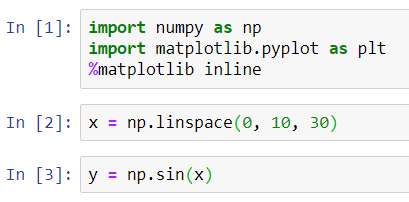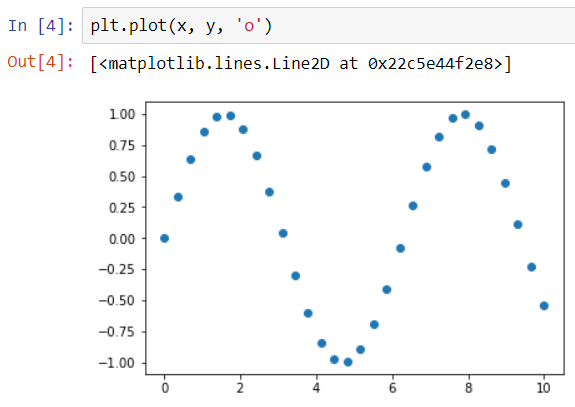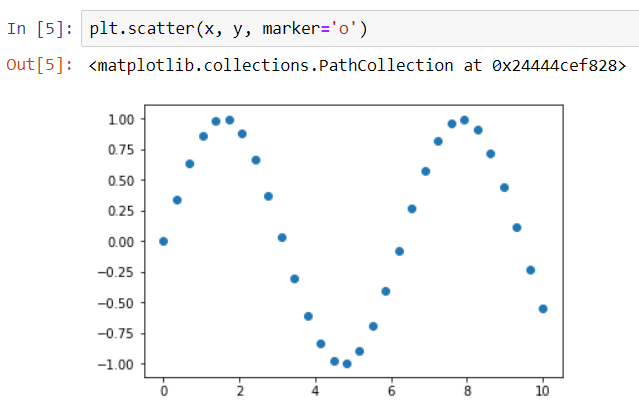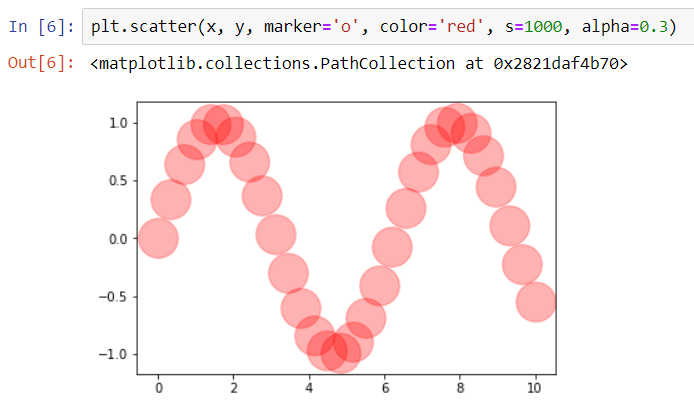Let us suppose that we have a set of coordinates and we want to plot them on the XY Plane. The plot which formed on the plane is termed as a Scatter Plot.

To build a scatter plot, Matplotlib provides us two functions-

• pyplot.plot(x, y, ‘<Marker>’)
• pyplot.scatter(x, y, marker=’<Marker>’)

Here, the marker is a symbol with which we want to represent each coordinate on the XY Plane.

The several markers available in Matplotlib are ‘o’, ‘.’, ‘,’, ‘x’, ‘+’, ‘v’, ‘^’, ‘’, ‘s’, ‘d’.

The major difference between the pyplot.plot() and pyplot.scatter() functions is, pyplot.scatter() is a more powerful method of creating scatter plots because in this we can have more control over every single coordinate we are plotting on the plane.

### Python implementation of Scatter Plots

Let us begin by importing the Matplotlib library and create a set of (x,y) coordinates to be plotted.Here, ‘x’ is a set of 30 values ranging from 0 to 10. And correspondingly, ‘y’ is a sinusoidal function for every ‘x’.

Note: We have used the ‘o’ marker in the plots below. The several other markers available in Matplotlib are ‘.’, ‘,’, ‘x’, ‘+’, ‘v’, ‘^’, ‘’, ‘s’, ‘d’.

#### Scatter Plot using pyplot.plot()#### Scatter Plot using pyplot.scatter()pyplot.scatter() provides us with a lot of features, such as we can modify the size of the bullets representing coordinates, choose a color for every coordinate, change the opacity of any of the coordinates we want.

Given below is an illustration of these features.